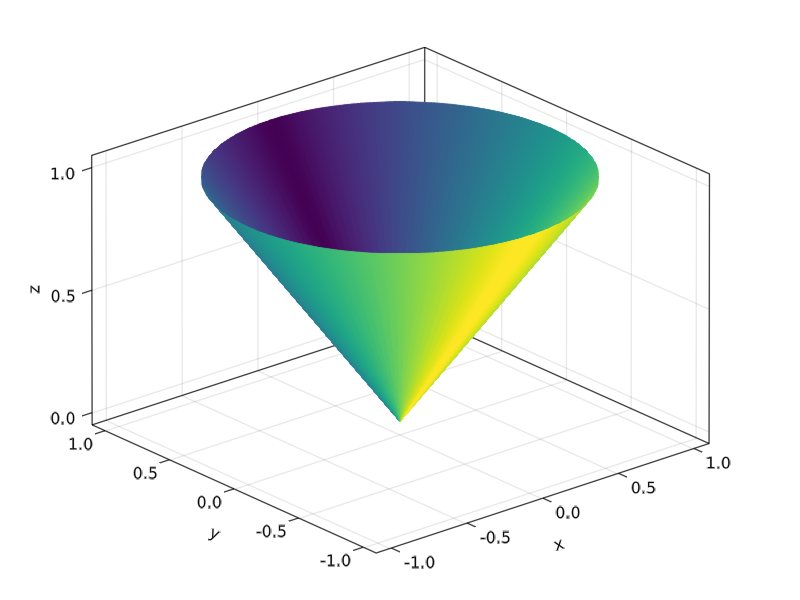# band

Makie.bandFunction
band(x, ylower, yupper; kwargs...)
band(lower, upper; kwargs...)


Plots a band from ylower to yupper along x. The form band(lower, upper) plots a ruled surface between the points in lower and upper.

Attributes

Available attributes and their defaults for Combined{Makie.band, T} where T are:

  ambient         Float32[0.55, 0.55, 0.55]
color           :black
colormap        :viridis
colorrange      MakieCore.Automatic()
cycle           [:color => :patchcolor]
diffuse         Float32[0.4, 0.4, 0.4]
inspectable     true
interpolate     false
lightposition   :eyeposition
linewidth       1
nan_color       RGBA{Float32}(0.0f0,0.0f0,0.0f0,0.0f0)
overdraw        false
shininess       32.0f0
specular        Float32[0.2, 0.2, 0.2]
ssao            false
transparency    false
visible         true
source

### Examples

using CairoMakie

f = Figure()
Axis(f[1, 1])

xs = 1:0.2:10
ys_low = -0.2 .* sin.(xs) .- 0.25
ys_high = 0.2 .* sin.(xs) .+ 0.25

band!(xs, ys_low, ys_high)
band!(xs, ys_low .- 1, ys_high .-1, color = :red)

fusing Statistics
using CairoMakie

f = Figure()
Axis(f[1, 1])

n, m = 100, 101
t = range(0, 1, length=m)
X = cumsum(randn(n, m), dims = 2)
X = X .- X[:, 1]
μ = vec(mean(X, dims=1)) # mean
lines!(t, μ)              # plot mean line
σ = vec(std(X, dims=1))  # stddev
band!(t, μ + σ, μ - σ)   # plot stddev band

fusing GLMakie
lower = fill(Point3f0(0,0,0), 100)
upper = [Point3f0(sin(x), cos(x), 1.0) for x in range(0,2pi, length=100)]
col = repeat([1:50;50:-1:1],outer=2)
band(lower, upper, color=col, axis=(type=Axis3,))